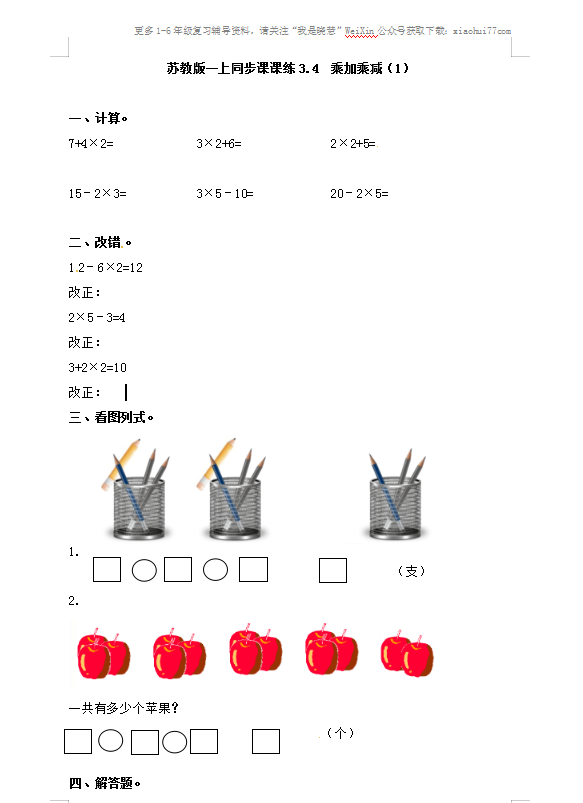7+4×2=             3×2+6=              2×2+5=

15－2×3=           3×5－10=            20－2×5=